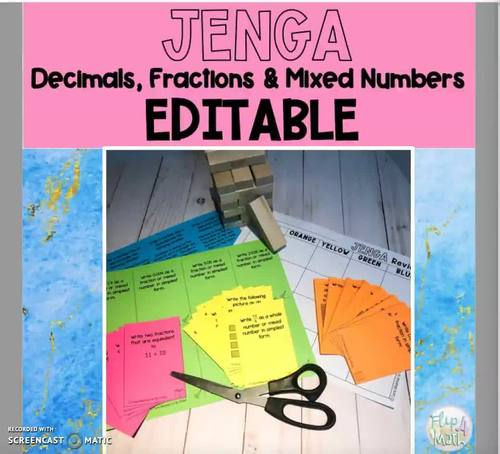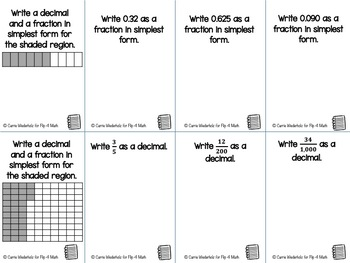# Decimals, Fractions and Mixed Numbers EDITABLE Jenga ActivitySubject
Resource Type
File Type

PDF

(6 MB|26 pages)
Product Rating
Standards
Also included in:
1. This resource includes both my Decimal, Fractions and Mixed Numbers Interactive Notebook Foldables resource as well as my Decimal, Fractions and Mixed Numbers EDITABLE Jenga Activity resource.
\$8.50
\$7.65
Save \$0.85
• Product Description
• StandardsNEW

By bringing JENGA into the classroom, the students will be engaged in the lesson as well as having fun with their classmates. This decimals, fractions and mixed numbers Jenga activity includes 8 task cards on problems involving fractions, decimals and mixed numbers for a total of 40 questions. The topics covered are:

1. Changing division problems to fractions and fractions to division problems

2. Write decimals as proper fractions and proper fractions as decimals

3. Mixed numbers and Improper Fractions

4. Write decimals as mixed numbers and write improper/mixed numbers as decimals (including repeating decimals)

5. Draw a number line to demonstrate a situation involving decimals

6. EDITABLE: Create your own fraction/decimal review card set

As an added bonus, I've made a set of cards EDITABLE which means that if you want to create other fraction/decimal problems you easily can through the use of Adobe Acrobat Reader.

*In order to do this activity, you need to have Jenga blocks (or any building blocks game) which you will have to color both ends with colored markers. You can also just buy the blocks already in different colors.

This product includes:

• Teacher prep set-up directions
• Teacher day of directions
• Jenga directions slide to present to students
• 40 decimal, fraction and mixed numbers playing cards
• Teacher Editable Cards Directions
• 8 EDITABLE fractions and decimals playing cards
• 3-, 4-, and 5-color work space sheets

Check out my other JENGA product:

Solving One-Step Equations EDITABLE JENGA Activity

Let's Connect

Blog

Instagram

Email: flip4mathteacher@gmail.com

Make sense of problems and persevere in solving them. Mathematically proficient students start by explaining to themselves the meaning of a problem and looking for entry points to its solution. They analyze givens, constraints, relationships, and goals. They make conjectures about the form and meaning of the solution and plan a solution pathway rather than simply jumping into a solution attempt. They consider analogous problems, and try special cases and simpler forms of the original problem in order to gain insight into its solution. They monitor and evaluate their progress and change course if necessary. Older students might, depending on the context of the problem, transform algebraic expressions or change the viewing window on their graphing calculator to get the information they need. Mathematically proficient students can explain correspondences between equations, verbal descriptions, tables, and graphs or draw diagrams of important features and relationships, graph data, and search for regularity or trends. Younger students might rely on using concrete objects or pictures to help conceptualize and solve a problem. Mathematically proficient students check their answers to problems using a different method, and they continually ask themselves, "Does this make sense?" They can understand the approaches of others to solving complex problems and identify correspondences between different approaches.
Understand a rational number as a point on the number line. Extend number line diagrams and coordinate axes familiar from previous grades to represent points on the line and in the plane with negative number coordinates.
Interpret a fraction as division of the numerator by the denominator (𝘢/𝘣 = 𝘢 ÷ 𝘣). Solve word problems involving division of whole numbers leading to answers in the form of fractions or mixed numbers, e.g., by using visual fraction models or equations to represent the problem. For example, interpret 3/4 as the result of dividing 3 by 4, noting that 3/4 multiplied by 4 equals 3, and that when 3 wholes are shared equally among 4 people each person has a share of size 3/4. If 9 people want to share a 50-pound sack of rice equally by weight, how many pounds of rice should each person get? Between what two whole numbers does your answer lie?
Total Pages
26 pages
Included
Teaching Duration
N/A
Report this Resource to TpT
Reported resources will be reviewed by our team. Report this resource to let us know if this resource violates TpT’s content guidelines.